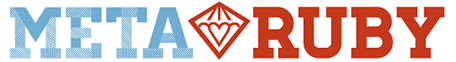# If array slot has no character value (a-z) then break

Hello, I am writing a simple program that takes a one line input of characters and ‘<’ and then outputs what the input would look like if the ‘<’ represented a desired backspace. For example if the input was “hello<<” then output should be “hel”. If the input is “helle<o my name is mm<att” the output should be “hello my name is matt”. My code does what I want but I think if I add a break statement saying if input[i] does not equal a character or ‘<’ symbol then break it would be faster. This might not do it so I am open to another way that you think would be a lot faster, maybe using a regex. The input can be up to 10^6 characters and must run in <1sec. I split the code, delete the backspaces and characters infront of the backspaces then re-join at the end. There has to be a quicker way…

Thanks for the help!

Here is my code so far

#!/usr/bin/ruby

``````i = 0
input = gets.chomp.split("")
length = input.length
loop do
``````
``````		if input[i]	== '<'
``````
``````			input.delete_at(i-1)
``````
``````			input.delete_at(i-1)
``````
``````			i = i -2
end
``````
``````			i+=1
``````
``````		if i == length # or... input[i] has no character in it.
``````
``````		break
``````
``````		end
end
``````
``puts "#{input.join("")}"``

An easier solution than what you are trying to do, would be to just look for `X<` and replace it with nothing.

Something along the lines of

``````def backspace(str)
result = str
while result.include?("<")
result.gsub!(/[^<]</, "")
end
result
end
``````

(untested)

1 Like

Yep, that works. Now I just have to make a case where if there is only “<” left it needs to break the loop. Or else the input hello<<<<<<<<<<< would run forever. Or any input that has more backspaces than characters.

Thanks for the help!

How would I say that?

psuedo…

if (result does not include word character)
break
end

You can just use the same code, but change it to:

``````def backspace(str)
result = str
while result[/[^<]</]
result.gsub!(/[^<]</, "")
end
result
end
``````

The `while result[/[^<]</]` line says: "While I can match a symbol, that is not `<`, followed by a `<`, I’ll continue. Then inside the while-loop, we replace that matched thing with nothing, and ask again.

Now it works for `a<<`, and will return `<`. If you want it to just return an empty string, you’ll need to replace the last line with: `result.gsub(/</, "")`.

2 Likes

Okay, I see. It must be getting stuck in an infinite loop or something… because test 4/20 says it exceeded the 1 second time limit but it seems like it would be quick. It’s probably inputting 10^6 characters. But still I would think this would execute faster unless its getting stuck

I modified the code a little to say if a word character is before ‘<’ then replace, thinking that would also save some time. This small problem is being a pain. I won’t bother you anymore you have helped enough.

here’s what I tried…

`````` #!/usr/bin/ruby

input = gets.chomp
while input[/[\w]</]
input.gsub!(/[\w]</, "")
end
input.gsub!(/</, "")
puts"#{input}"``````